### Linear Regression in Excel

The linear regression is similar to multiple regression. But, there are difference between them. We’d perform the task that together, in a step-by-step format. But, first you’d need to get the Data Analysis by following through these steps: file > options > add-ins> go > data analysis > ok. Further, you’d also needs a data that look like this: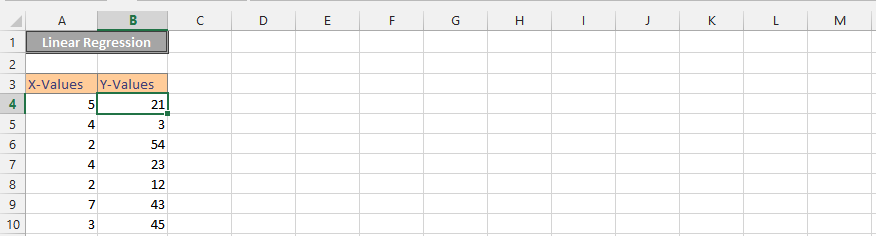1. Click on Data (1), and then press on the Data Analysis (2).2. Click on the regression.3. Select the cells for y-values (1), then the ones for x-values (2), and then check the labels (3). Finally, press ok (4).4. Click on an empty cell (1), and then press insert (2). Press scatter chart (3), and choose a scatter chart (4).5. Right click on the chart (1), and choose Select Data (2).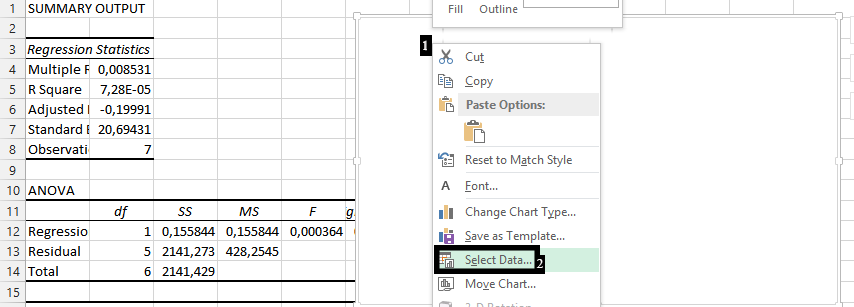7. Write the series name (1), choose the cells for X-values (2), and for Y-values (3). Press ok (4).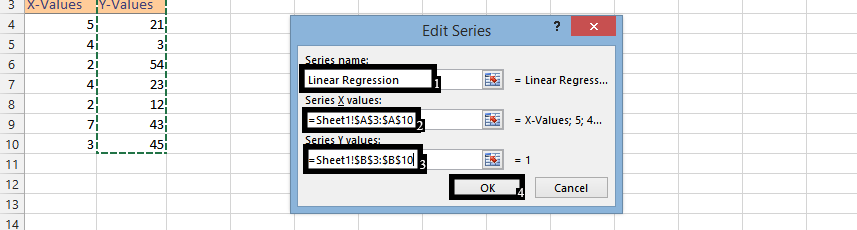Note: The values are the data, which would be in another spreadsheet.

8. Click Ok.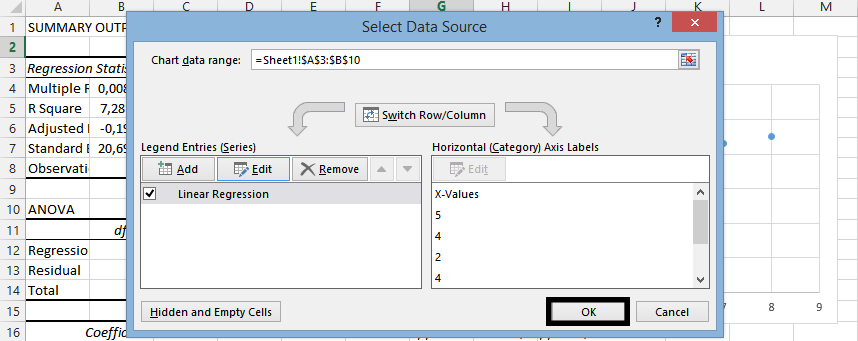9. Right Click on any of the markers (1), and then select add trendline (2).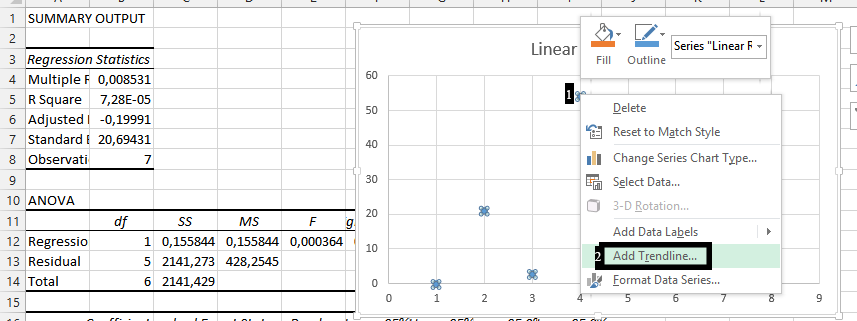10. If you would prefer, check the Display Equation on Chart (1), and the R-square value on chart (2).In conclusion, you should have done a linear regression that looks like this:## Template

```Further reading: